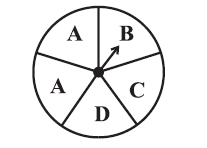# vs.eyeandcontacts.com

## Chapter 5 Data Handling Exercise 5.3

Question 1: List the outcomes you can see in these experiments.
(a) Spinning a wheel
(b) Tossing two coins togethera) There are four letters A, B, C and D in a spinning wheel. Therefore, there are 4 outcomes.

b) When two coins are tossed together, we get four possible outcomes - HH, HT, TH, TT.

Question 2: When a die is thrown, list the outcomes of an event of getting
(i) (a) a prime number (b) not a prime number.
(ii) (a) a number greater than 5 (b) a number not greater than 5.

i)
a) Outcomes of event of getting a prime number are 2, 3 and 5.

b) Outcomes of event of not getting a prime number are 1, 4 and 6.

ii)
a) Outcomes of event of getting a number greater than 5 is 6.

b) Outcomes of event of not getting a number greater than 5 are 1, 2, 3, 4 and 5.

Question 3: Find the
(a) Probability of the pointer stopping on D in (Question 1-(a))?
(b) Probability of getting an ace from a well shuffled deck of 52 playing cards?
(c) Probability of getting a red apple. (See figure below)a)
Number of pointers in spinning wheel = 5
Number of sector where the pointer stops = 1 (D)
Probability of pointer stopping at D = 1/5

b)
Number of aces in deck = 4
Total number of playing cards = 52
Probability of getting an ace = 4/52 = 1/13

c)
Total number of apples = 7
Total number of red apples = 4
Probability of getting red apple = 4/7

Question 4: Numbers 1 to 10 are written on ten separate slips (one number on one slip), kept in a box and mixed well. One slip is chosen from the box without looking into it. What is the probability of
(i) getting a number 6?
(ii) getting a number less than 6?
(iii) getting a number greater than 6?
(iv) getting a 1-digit number?

i)
Number of slips = 10
Probability of getting number 6 in slip = 1
Probability of getting slip = 1/10

ii)
Number of slips = 10
Probability of getting number less than 6 in slip = 5 (1, 2, 3, 4, 5)
Probability of getting slip less than 6 = 5/10 = 1/2

iii)
Number of slips = 10
Probability of getting number greater than 6 in slip = 4 (7, 8, 9, 10)
Probability of getting slip greater than 6 = 4/10 = 2/5

iv)
Number of slips = 10
Probability of getting number with one-digit in slip = 9 (1, 2, 3, 4, 5, 6, 7, 8, 9)
Probability of getting slip with one-digit number = 9/10

Question 5: If you have a spinning wheel with 3 green sectors, 1 blue sector and 1 red sector, what is the probability of getting a green sector? What is the probability of getting a non-blue sector?

Total number of sectors = 5
Number of sectors that are green = 3
Probability of getting green sectors = 3/5

Total number of sectors = 5
Number of sectors that are non-blue = 4
Probability of getting non-blue sectors = 4/5

Question 6: Find the probabilities of the events given in Question 2.

i)
a)
Number of prime numbers = 3 (2, 3, 5)
Total number of outcomes = 6
Probability of getting a prime number = 3/6 = 1/2

b)
Number of non-prime numbers = 3 (1, 4, 6)
Total number of outcomes = 6
Probability of getting a non-prime number = 3/6 = 1/2

ii)
a)
Numbers greater than 5 = 1 (6)
Total number of outcomes = 6
Probability of getting a prime number = 1/6

b)
Numbers less than 5 = 5 (1, 2, 3, 4, 5)
Total number of outcomes = 6
Probability of getting a non-prime number = 5/6